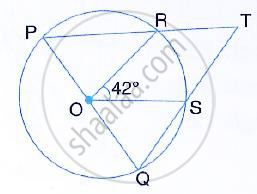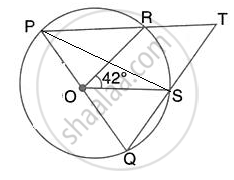Share

In the Given Figure, Pq is the Diameter of the Circle Whose Centre is O. Given ∠Ros = 42°, Calculate ∠Rts. - ICSE Class 10 - Mathematics

ConceptArc and Chord Properties - the Angle that an Arc of a Circle Subtends at the Center is Double that Which It Subtends at Any Point on the Remaining Part of the Circle

Question

In the given figure, PQ is the diameter of the circle whose centre is O. Given ∠ROS = 42°, Calculate ∠RTS.SolutionJoin PS.
∠PSQ = 90°
(Angle in a semicircle)
Also, ∠SPR=1/2 ∠ROS
(Angle ate the centre is double the angle at the circumference subtended by the same chord)
⇒ SPT =1/2 xx 42°= 21°
∴ In right triangle PST,
∠PTS = 90° -∠SPT
⇒ ∠RTS = 90°- 21° = 69°

Is there an error in this question or solution?

Video TutorialsVIEW ALL 

Solution In the Given Figure, Pq is the Diameter of the Circle Whose Centre is O. Given ∠Ros = 42°, Calculate ∠Rts. Concept: Arc and Chord Properties - the Angle that an Arc of a Circle Subtends at the Center is Double that Which It Subtends at Any Point on the Remaining Part of the Circle.
S